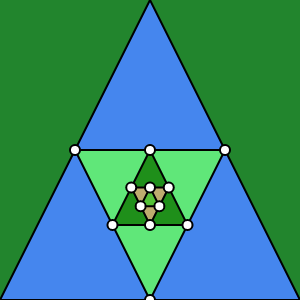# Triangle Inset

example p5.js javascript input

This sketch draws a triangle inside a triangle inside a triangle inside a triangle inside a triangle.# Remix Ideas

• Try alternating shapes: draw a square inside a triangle inside a circle.
• Give each individual section of each triangle a different color (3 sections per triangle).
• Instead of using the midpoint of each line, try moving the next point closer to one of the previous points.